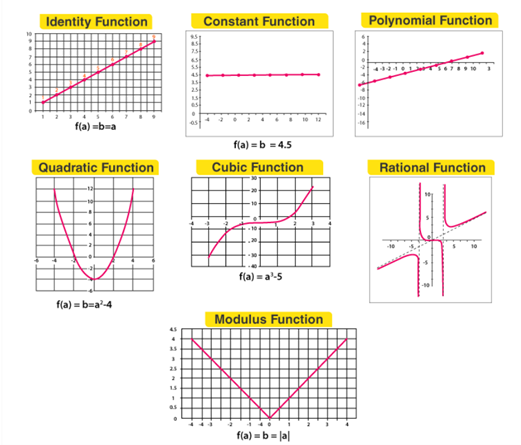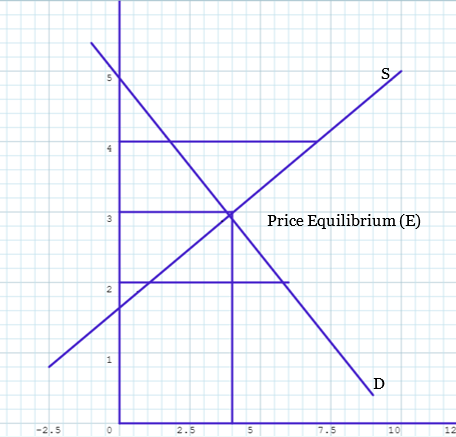top of page
Search

# Functions are in Business Studies

According to Nykamp DQ, a technical definition of a function is a relation from a set of inputs to a set of possible outputs where each input is related to exactly one output. It can be said that a function is an expression, rule, or law which describes a relationship between two variables where one is an independent variable and another is a dependent variable.A function can be used in a business situation where a particular variable (e.g. cost, demand) depends upon certain other variables (e.g. quantity of output produced, price). In decision-making, the first requirement for the use of a function is to identify the relevant factors also called variables in the problem, and interrelationships between them. A basic function can be formulated like y= ʄ (x) where ‘y’ depends upon ‘x’. In this function, ‘y’ is the dependent variable and ‘x’ is the independent variable.Using a function a business can be able to estimate the probable impact of a decision. A business can also be able to compare projects to prioritize the beneficial project while using the functions in the decision-making process of the business.

## What is Function?

The formal definition of a function is given a set of inputs ‘X’ also called ‘domain’ and a set of possible outputs ‘Y’ called ‘codomain’ as a set of ordered pairs (x,y) where x Є X and y Є Y, subject to the restriction that there can be only one ordered pair with the same value of x. It can be stated that ʄ is a function from X to Y using the function notation ʄ:X→Y.

The modern definition of function was first defined by the German mathematician Peter Dirichlet in 1837. According to Peter Dirichlet,

“If a variable y is so related to a variable x that whenever a numerical value is assigned to x, there is a rule according to which a unique value of y is determined, then y is said to be a function of the independent variable x.”

## Types of Functions

There are various types of functions in mathematics. The frequently used functions are as follows:## Functions are in Business and Economics

The situation where one quantity decides another are called functions. For instance, an amount of \$5,000 is invested at an annual rate of 7.25%, and the return of the invested amount is a function of the length of time the money is invested. In a business, functions are important tools to make a decision. Let’s have a look into the application of functions in a business.

Let’s assume a business firm produces only one product. ‘Q’ is the amount of that product or output and ‘C’ is the cost of production. Therefore, the function of the product will be C= ʄ(Q) which indicates the total costs (C) of the business depends on the amount of output produced (Q). On the other hand, the revenue (R) of the business firm depends upon the output (Q). Therefore, the function for the business firm will be R= ʄ(Q). As a result, the profit (Π) may be derived by the function using Profit= Revenue – Cost.

`Π = R – C`

The frequently used functions in business and economics are as follows:

### Linear function:

The function in the form of ax + b is called linear function, where a, b ∈ R & a ≠ 0. The graph will be a straight line.The functions in the form of y = ax2 + bx + c where a, b, c ∈ R, a ≠ 0 will be known as Quadratic function. The graph will be parabolic.### Polynomial function:

A real-valued function f : P → P defined by y = ʄ(a)=h0+h1a+…..+hnan where n ∈ N, and h0+h1+…..+hn ∈ P, for each a ∈ P, is called polynomial function.

• N = a non-negative integer.

• The degree of the Polynomial function is the highest power in the expression.

• If the degree is zero, it’s called a constant function.

• If the degree is one, it’s called a linear function. Example: b = a+1.

• Graph type: Always a straight line.

So, a polynomial function can be expressed as :

`ʄ (x)= anxn+an-1xn-1+…..+a1x1+a0`

### Rational function:

These are the real functions of the type ʄ(a)/g(a) where ʄ(a) and g(a) are polynomial functions of a defined in a domain, where g(a) ≠ 0.

For example ʄ : P – {– 6} → P defined by ʄ(a) = ʄ(a+1)/g(a+2), ∀a∈P – {–6 }is a rational function.

Graph type: Asymptotes (the curves touching the axes lines).

In business and economics, an equilibrium is important to create a balanced market and an efficient market as well as the break-even analysis. The equilibrium and the break-even analysis can be calculated using functions.## Equilibrium market

The market equilibrium of a product is a situation where supply and demand are balanced. The graphs for supply and demand curves have a price on the vertical axis and quantity on the horizontal axis and therefore the supply curve and the demand curve for a particular product or service can appear on the same graph. The situation where the supply curve and the demand curve intersects is called the price equilibrium of that product or service. It is assumed that the market of that particular product or service will operate at this price.In the above graph, the supply curve (S) and the demand curve (D), both intersect at the point of equilibrium (E), with the equilibrium quantity of 4 and with an equilibrium price of 3. At the equilibrium situation, the demand for the good equals the supply of that good. When the price is 2, the quantity demanded exceeds the quantity supplied, therefore there is excess demand. On the other hand, when the price is 4, the quantity supplied exceeds the quantity demanded, therefore there is excess supply.

The price equilibrium of the good can also be expressed in a table.

Price (P) Quantity supplied (Qs) Quantity demanded (Qd)

0 -5 10

1 -2 8

2 1 6

3 4 4

4 7 2

5 10 0

The equilibrium price in the table is 3 which is indicated in the graph as ‘E’. The equilibrium price can be calculated using functions. Let’s solve the equilibrium using functions.

Demand function, Qd = a – bP

Let’s solve for ‘b’, which is the slope of the demand curve.

b = (6-8)/(2-1) = -2

Let’s find the ‘a’, which is the vertical intercept.

a = 10

So, the demand function is Qd = 10-2P

Supply function, Qs = c + dP

Let’s solve for ‘d’, which is the slope of the demand curve.

d = (10-7)/(5-4) = 3

Let’s find the ‘c’, which is the vertical intercept.

c = -5

So, the demand function is Qs = -5+3P

To solve the equilibrium price, the function will be the supply function equal to the demand function.

Qs = Qd

-5+3P = 10-2P

P = 3

Qs = -5+3P = -5+3(3) = 4 = Qd

## References

1. Nykamp DQ, “Function definition.” From Math Insight. http://mathinsight.org/definition/function Accessed 13 August 2021.

2. Britannica, The Editors of Encyclopaedia. "function". Encyclopedia Britannica, 4 Jun. 2021, https://www.britannica.com/science/function-mathematics. Accessed 13 August 2021.

3. https://byjus.com/jee/functions-and-its-types/ Accessed on August 15, 2021.

4. https://www.khanacademy.org/economics-finance-domain/microeconomics/supply-demand-equilibrium/market-equilibrium-tutorial/a/market-equilibrium Accessed on August 15, 2021.

5. Dowling, Edward T. (2001). Introduction to Mathematical Economics, Third Edition. (Schaum's outline series.)